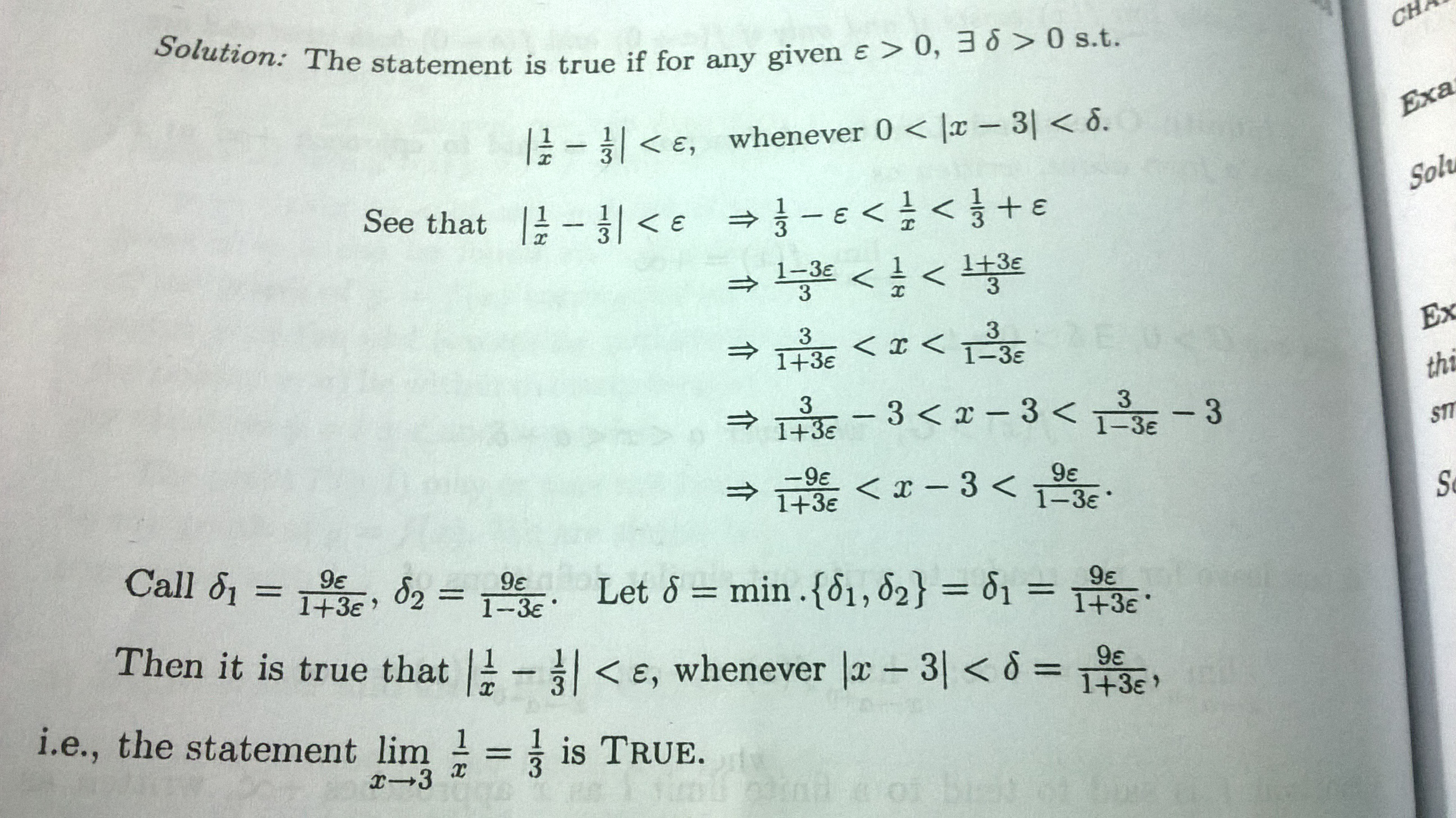# Faults in epsilon-delta proof?Above is the textbook proof that $\lim\limits_{x\to 3}\frac 1x=\frac 13$. I'm not sure if this is completely correct or not since I noticed that some of the implied inequalities doesn't hold $\forall~\epsilon\gt 0$. Take, for example, the inequality in the 4th line of the image :

$$\frac 3{1+3\epsilon}\lt x\lt \frac 3{1-3\epsilon}$$

that is implied by the 3rd line. That inequality doesn't hold, for say $\epsilon=2\gt 0$, i.e., there is no real $x$ for when $\epsilon=2$ that satisfies that inequality.

Also, I wonder why they took the $\min$ of $\delta_1$ and $\delta_2$ to be the $\delta$ since I think we should take the $\max$. This is because $-a\lt x\lt b\implies |x|\lt \max(a,b)~\forall~a,b\in\Bbb R$.

I'm pretty much a beginner at rigorous stuff like this, so I might be completely wrong in my thinking. I look forward to helpful responses from the community. Thanks.

• I would think the inequality should flip at that point, i.e. $\frac{1}{2}<x<3\Rightarrow 2>\frac{1}{x}>\frac{1}{3}$ – Craig Nov 17 '15 at 5:38
• There is nothing wrong with that inequality. If $\epsilon = 2$ you can take $x=1$ for example. – R_D Nov 17 '15 at 5:52
• In order to arrive at the inequality in question, the author tacitly assumes that $1-3\epsilon>0 \implies \epsilon <\frac13$. – Mark Viola Nov 17 '15 at 5:58
• The textbook tries to focus on obtaining an expression for $\delta$ in terms of $\epsilon$ using algebraic manipulation of the target inequality $|1/x - 1/3| < \epsilon$ and @Dr.MV has rightly pointed out when this technique will fail. The proper approach is the one given in Gudson Chou's answer which tries to simplify the inequality altogether by removing $x$ from denominator. – Paramanand Singh Nov 17 '15 at 6:15
• @Craig, the inequality was indeed flipped. $a\lt b\lt c\implies \frac 1c\lt\frac 1b\lt\frac 1a$ – learner Nov 17 '15 at 9:27

If $x \neq 0$, then $$\bigg| \frac{1}{x} - \frac{1}{3} \bigg| = \frac{|x-3|}{3|x|};$$ if $|x-3| < 1$, then $||x| - 3| \leq |x-3| < 1$ by triangle inequality, implying that $2 < |x|$, implying that $$\frac{|x-3|}{3|x|} < \frac{|x-3|}{6};$$ given any $\varepsilon > 0$, we have $|x-3|/6 < \varepsilon$ if $|x-3| < 6\varepsilon$. Putting all the previous things together, we conclude that, for every $\varepsilon > 0$, if $x \neq 0$ and if $|x-3| < \min \{ 1, 6\varepsilon \}$ then $$\bigg| \frac{1}{x} - \frac{1}{3} \bigg| < \varepsilon.$$
• Thanks, but I think there's a typo in your answer. Shouldn't it be $\min\{1,6\epsilon\}$ which is to be taken as $\delta$ ? – learner Nov 17 '15 at 9:47